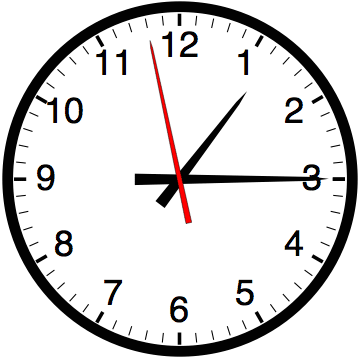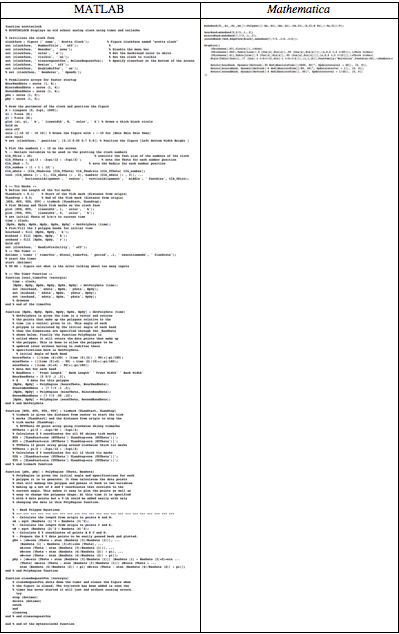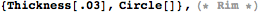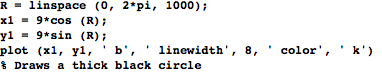Browse by Topic
Related Topics

# Always the Right Time for Mathematica…

I was rummaging around on the web the other day and ran across an example of implementing an analog clock, written in MATLAB—a numerical matrix system that’s sometimes compared to the numerics component of Mathematica.

I was curious to see how the MATLAB implementation compares to a Mathematica implementation, so I took a few minutes to write its equivalent.

Here’s a quick shot of part of the result (download the Mathematica notebook to see the clock run in real time):The exercise surprised me—not because the Mathematica code is so concise and straightforward, but because of how much I’d have had to learn and how hard I’d have had to think and what volume of code I’d have had to write to do the same thing with MATLAB. After a statement like that in our company blog, I can hear you thinking that the spin doctors are hard at work. But have a look for yourself…

Here’s the original MATLAB version on the left, and the equivalent Mathematica version on the right (click the code below to see it at a readable size):The MATLAB source is about 90 lines, if you don’t count the comments. I’ve included the original comments in the MATLAB example above, which admittedly increases its apparent volume. But because of the cryptic nature of the MATLAB code, I’d say the comments are a necessary part of the source.

I feel almost silly adding a comment to explain that this Mathematica code draws the thick black rim of the clock.But it would be downright irresponsible not to explain in a comment what the corresponding snippet of MATLAB code does:Of course, volume of code is not an entirely fair measure of the ease or difficulty of writing a program. Code written in APL or Perl tends to be compact, but it is also generally undecipherable. The Mathematica clock code is not only short—it’s easy to conceive and easy to read and understand. Decide for yourself from this line-by-line code comparison whether you’d rather implement the clock using MATLAB or Mathematica.

To be completely up front about this comparison, I don’t know whether the MATLAB code’s author is a novice or a crack MATLAB programmer. I, on the other hand, have been using Mathematica for many, many years.

I suspect that the author is at least a moderately sophisticated MATLAB user, or he wouldn’t know about timers and double-buffering and catching exceptions and such arcana that are necessary to make the MATLAB clock work well.

And although I am an experienced Mathematica programmer, I didn’t have to draw much on that experience to write the clock. I was able to set down the code more or less as I conceived it, without having to take detours to puzzle out technological programming details. The code you see above is the unpolished, unrefined first version of the code as I wrote it while looking at the MATLAB source and a screen capture of the appearance of the clock.

It took roughly five minutes from start to finish. I could explain it to my mother in another ten.

There are lots of other Mathematica niceties that make implementing and using the clock a joy. Evaluating the clock code immediately gives a running clock in the output cell below the code. That immediate feedback is a huge help when you’re writing code. For instance, to get the clock ticks positioned and sized right, I made a guess at initial values and then quickly refined them to look like the MATLAB screenshot by tweaking and re-evaluating a few times.

And Mathematica is careful not to waste your CPU resources, even though you may have hundreds of dynamic objects like the clock in a notebook.

When I scroll the clock off screen, Mathematica takes notice and doesn’t spend any time updating it. When I scroll it back on screen (in Mathematica mind you, not the short Flash above), it continues at the correct time, giving the appearance of having been running all the while. Amazingly, if I were to close the notebook and send it to Shanghai, the clock would still be running and showing the correct time when the notebook was opened there.

You may be thinking that I chose the clock as a particular example that is easy to implement with Mathematica and difficult with MATLAB. I didn’t. I just happened to run across the clock and was curious how the implementations compare.

The ease of using Mathematica is the result of 20 years of careful, integrated design and implementation of things like symbolics, numerics, graphics and dynamic behavior. It’s the way all the pieces fit nicely together that shortens the distance from your ideas to their interactive implementations.

But you don’t have to take my word for it. There are plenty of examples of how intriguing ideas can easily be explored with a minimum of Mathematica code at the Wolfram Demonstrations Project. It’s no coincidence that so many people on this blog keep referencing it. There are just so many different applications on the site (just over 1500 at last count). Get yourself Mathematica Player and check it out.